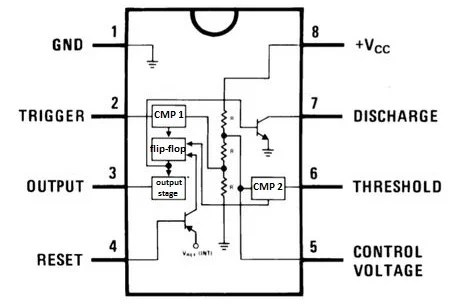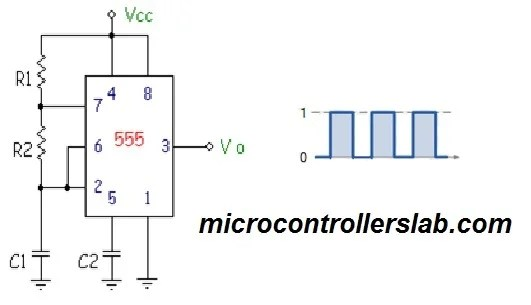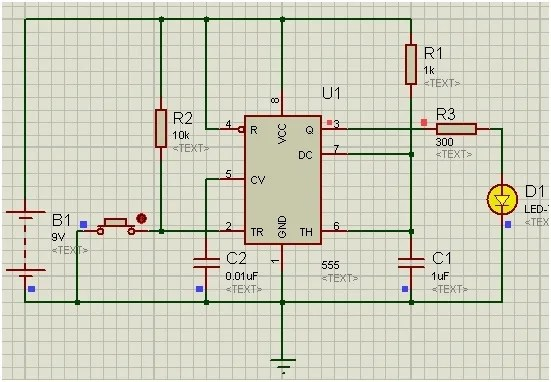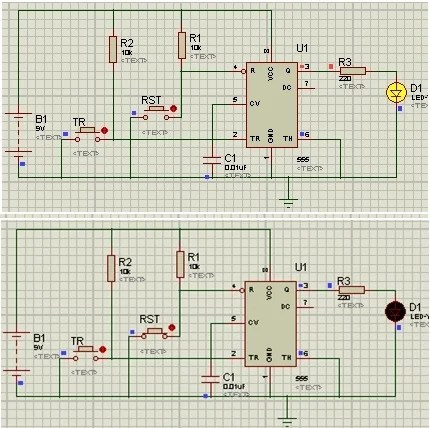# 555 timer in different modes of operation with circuit diagram

555 TIMER Timers, as the name specified, are the electronics circuits used for measuring time intervals. In this article we will cover about 555 timer. This integrated circuit can be used in variety of ways from which the basic one is to produce accurate and stable delays in electronic circuits. It is available in 8 pin DIP and 14 pin DIP. 555 timers are very popular in electronics projects.  Some they serve the purpose of microcontroller and used instead of microcontroller to avoid from microcontroller based project.

### Pin out of 555 timer### FEATURES of 555 timer

It is used as

Other important features are:

• Wide range of power supply (5-18)V
• Sinking High current output (200mA of load current)
• Variable duty cycle
• Logic compatible trigger and reset inputs
• High temperature stability

### INTERNAL CIRCUIT of 555 timer

The internal circuit of this 555 timer consists of comparators, flipflop, transistors, resistors and output stage.RESISTOR:              The three resistors used in it are of 5Kohm and they served as voltage divider between Vcc and ground. All resistors are of same value, so the voltage at the resistors junction is 2/3 Vcc and 1/3 Vcc which is used as reference voltage for the comparators.

COMPARATOR:     It is a circuit that compares an input with a reference voltage (whether input is higher or lower voltage than reference) and outputs a low or high signal. Many transistors are used for this comparator purpose. Trigger is the inverting input to the comparator 1. Threshold is the non-inverting input to the comparator 2. The comparator 1 connectedto pin 2 compares the trigger input to a reference voltage of 1/3 of Vcc. The comparator 2 connected to pin 6 compares the threshold input to a reference voltage of 2/3 of Vcc.

FLIP FLOP:              It is a circuit that can be in one of two states based on the state of inputs. The two comparators outputs are the inputs of flip-flop.  When trigger comparator outputs a low signal (whatever the output of threshold comparator), the flip flop switches to high output. When trigger and threshold, both comparators are outputting a high signal, the flip flop switches output to low output. Time of high pulse output can be manually reset by pulsing the reset pin to low.

TRANSISTORS:      Two transistors are also shown in figure. One transistor is N-P-N and its collector is connected to pin 7. This configuration is known as open drain or open collector. Usually this pin is connected to a capacitor and is used to discharge the capacitor each time the output pin goes low. Second transistor is P-N-P which is connected to pin 4. The purpose of this transistor is to buffer the reset pin and so the 555 IC does not source current from this pin and cause it to sag in voltage.

OUTPUT STAGE:   It acts as a buffer between the 555 timer and the loads that may be attached to its output pin. This stage supplies current to the output pin.

### BASIC OPERATIONs of 555 timer

By wiring 555 timer with resistors and capacitors in different ways, It can operate in 3 modes:

1. Monostable mode
2. Astable mode
3. Bistable mode

### Modes of 555 timer

MONOSTABLE MODE:This mode is used for creating time delays. In this mode the 555 timer outputs a high pulse.A monostable is a single stable state, i-e the off state. Whenever it is triggered by an input pulse, the monostable switches to its temporary state. It remains in that state for a period of time which is determined by an RC network. It then returns to its stable state.

• The trigger pin is set low (less than 1/3Vcc). Comparator connected to the trigger pin will have low output and sets the state of flip flop, changing output from low to high.
• Discharge transistor connected to pin 7 will get off. There will be no short circuit across capacitor. Charge will build up across the capacitor until voltage across the capacitor equals 2/3 of the supply voltage (Pin 6 voltage= 2/3 Vcc).
• Now at this point, the comparators output goes “HIGH. This will resets the flip-flop back to its original state (low).
• Now transistor gets ON and discharges the capacitor to ground through pin 7. This causes the output to change its state back to the original stable (low).
• The output remains low until the trigger pin is pulsed low again.
• The amount of time that the output voltage remains “HIGH” or at a logic “1” level is given by the following time constant equation.

ASTABLE MODE:This mode outputs an oscillating pulse signal. The output switches between high and low states at a tunable frequency and pulse width. Frequency depends on the values of the two resistors (R1 and R2) and capacitor C1. Trigger pin is connected to the threshold pin which causes the output to continuously toggle between the high and low states.

Frequency of Output = 1/[0.7*(R1+2*R2)*C1]

duty cycle = (R1+ R2) / (R1 + 2*R2)

• Initially, there is no charge on the capacitor C1, so the voltage across the capacitor is zero. Since capacitor C1, Threshold pin and Trigger pin are connected at same, so these both will also be at zero volts. This drives the output high.
• Vcc is applied, the current flowing through resistors start to accumulate charge on the capacitor C1. This causes the voltage across the capacitor C to increase.
• When the voltage across the capacitor C1 equals 2/3Vcc, it makes the threshold pin high, this flips the comparator attached to the threshold pin. This drives the output low and enables the discharge pin.
• When the discharge is pin enabled, the capacitor will discharge through R2.
• Once the voltage across the capacitor C1 equals 1/3Vcc, it makes the the trigger pin low. This flips the comparator attached to the trigger pin and drives the output high. By repeating above steps, the output switches between the high and low states to produce a continuous pulse wave.

BISTABLE MODE:This mode causes the 555 timer to toggle its output between high and low states depending on the state of two inputs. The circuit is stable in both states. It remains in the same state (either HIGH or LOW) until an external trigger is applied.

• In this mode, there is no RC network like the other two modes of 555, hence there are no equations.
• This circuit is actually a flip-flop.
• The two inputs are the trigger (Pin 2) and reset pins (Pin 4).
• By default, both are kept high by pull up resistors in bistable mode.
• When the trigger pin is pulsed low, (less than 1/3(Vcc)), it gives high output. The output will remain high even if the trigger pin is set high again.
• When the reset pin is pulsed low, the output goes low. The output will remain in this state even if the reset pin goes high again.

### PROTEUS SIMULATIONS of 555 timer

Example 1:

Here, we are using 555 timer in monostable mode. When trigger pin is set low, it gives high output and then output goes low.Example 2:

Here, we are using 555 timer in astablemode. When trigger pin is set low, it gives high and low output pulses.Example 3:Here, we are using 555 timer in Bistable mode. When trigger pin is set low, it gives high output and will remain in that state until the reset button is pressed. When reset is pressed it gives low output. Again we can make the output high by pressing trigger button.